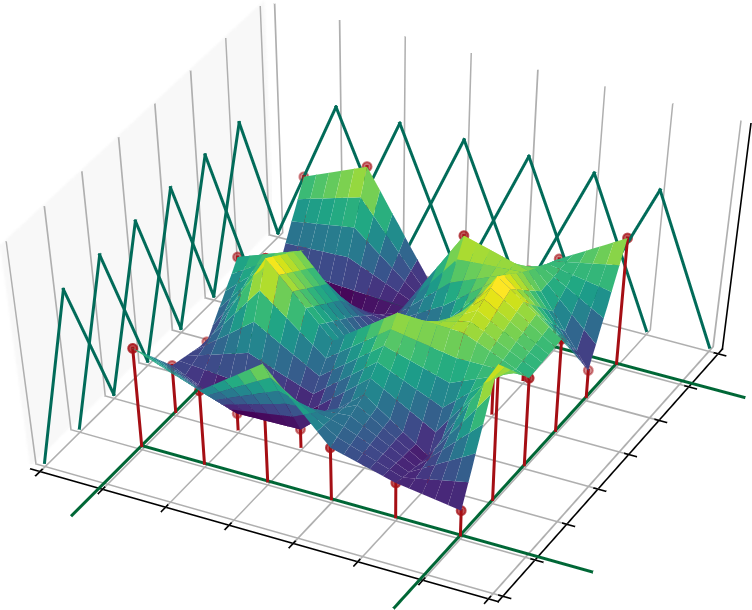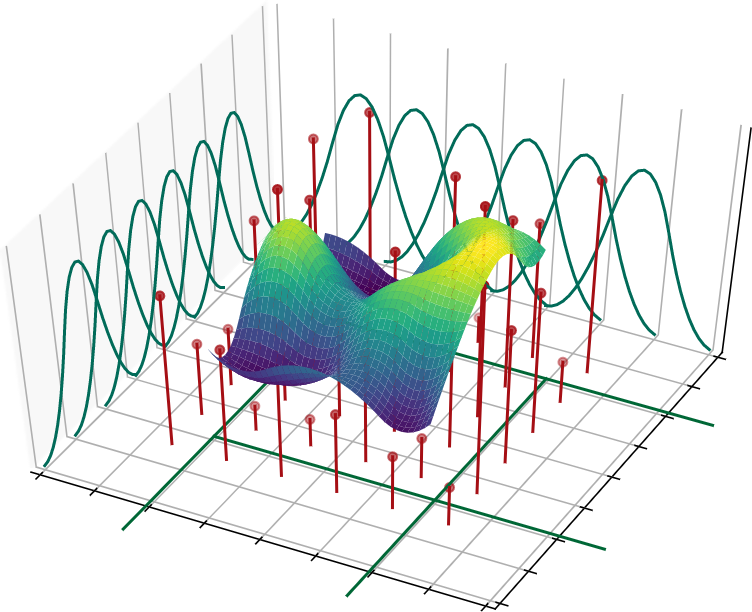Implementation of the Spline-Based Convolution Operator of SplineCNN in PyTorch

# Spline-Based Convolution Operator of SplineCNN

This is a PyTorch implementation of the spline-based convolution operator of SplineCNN, as described in our paper:

Matthias Fey, Jan Eric Lenssen, Frank Weichert, Heinrich Müller: SplineCNN: Fast Geometric Deep Learning with Continuous B-Spline Kernels (CVPR 2018)

The operator works on all floating point data types and is implemented both for CPU and GPU.

## Installation

### Anaconda

Update: You can now install pytorch-spline-conv via Anaconda for all major OS/PyTorch/CUDA combinations 🤗 Given that you have pytorch >= 1.8.0 installed, simply run

conda install pytorch-spline-conv -c pyg


### Binaries

We alternatively provide pip wheels for all major OS/PyTorch/CUDA combinations, see here.

#### PyTorch 2.0

To install the binaries for PyTorch 2.0.0, simply run

pip install torch-spline-conv -f https://data.pyg.org/whl/torch-2.0.0+${CUDA}.html  where ${CUDA} should be replaced by either cpu, cu117, or cu118 depending on your PyTorch installation.

cpu cu117 cu118
Linux
Windows
macOS

#### PyTorch 1.13

To install the binaries for PyTorch 1.13.0, simply run

pip install torch-spline-conv -f https://data.pyg.org/whl/torch-1.13.0+${CUDA}.html  where ${CUDA} should be replaced by either cpu, cu116, or cu117 depending on your PyTorch installation.

cpu cu116 cu117
Linux
Windows
macOS

Note: Binaries of older versions are also provided for PyTorch 1.4.0, PyTorch 1.5.0, PyTorch 1.6.0, PyTorch 1.7.0/1.7.1, PyTorch 1.8.0/1.8.1, PyTorch 1.9.0, PyTorch 1.10.0/1.10.1/1.10.2, PyTorch 1.11.0 and PyTorch 1.12.0/1.12.1 (following the same procedure). For older versions, you need to explicitly specify the latest supported version number or install via pip install --no-index in order to prevent a manual installation from source. You can look up the latest supported version number here.

### From source

Ensure that at least PyTorch 1.4.0 is installed and verify that cuda/bin and cuda/include are in your $PATH and $CPATH respectively, e.g.:

$python -c "import torch; print(torch.__version__)" >>> 1.4.0$ echo $PATH >>> /usr/local/cuda/bin:...$ echo \$CPATH
>>> /usr/local/cuda/include:...


Then run:

pip install torch-spline-conv


When running in a docker container without NVIDIA driver, PyTorch needs to evaluate the compute capabilities and may fail. In this case, ensure that the compute capabilities are set via TORCH_CUDA_ARCH_LIST, e.g.:

export TORCH_CUDA_ARCH_LIST = "6.0 6.1 7.2+PTX 7.5+PTX"


## Usage

from torch_spline_conv import spline_conv

out = spline_conv(x,
edge_index,
pseudo,
weight,
kernel_size,
is_open_spline,
degree=1,
norm=True,
root_weight=None,
bias=None)


Applies the spline-based convolution operatorover several node features of an input graph. The kernel function is defined over the weighted B-spline tensor product basis, as shown below for different B-spline degrees.### Parameters

• x (Tensor) - Input node features of shape (number_of_nodes x in_channels).
• edge_index (LongTensor) - Graph edges, given by source and target indices, of shape (2 x number_of_edges).
• pseudo (Tensor) - Edge attributes, ie. pseudo coordinates, of shape (number_of_edges x number_of_edge_attributes) in the fixed interval [0, 1].
• weight (Tensor) - Trainable weight parameters of shape (kernel_size x in_channels x out_channels).
• kernel_size (LongTensor) - Number of trainable weight parameters in each edge dimension.
• is_open_spline (ByteTensor) - Whether to use open or closed B-spline bases for each dimension.
• degree (int, optional) - B-spline basis degree. (default: 1)
• norm (bool, optional): Whether to normalize output by node degree. (default: True)
• root_weight (Tensor, optional) - Additional shared trainable parameters for each feature of the root node of shape (in_channels x out_channels). (default: None)
• bias (Tensor, optional) - Optional bias of shape (out_channels). (default: None)

### Returns

• out (Tensor) - Out node features of shape (number_of_nodes x out_channels).

### Example

import torch
from torch_spline_conv import spline_conv

x = torch.rand((4, 2), dtype=torch.float)  # 4 nodes with 2 features each
edge_index = torch.tensor([[0, 1, 1, 2, 2, 3], [1, 0, 2, 1, 3, 2]])  # 6 edges
pseudo = torch.rand((6, 2), dtype=torch.float)  # two-dimensional edge attributes
weight = torch.rand((25, 2, 4), dtype=torch.float)  # 25 parameters for in_channels x out_channels
kernel_size = torch.tensor([5, 5])  # 5 parameters in each edge dimension
is_open_spline = torch.tensor([1, 1], dtype=torch.uint8)  # only use open B-splines
degree = 1  # B-spline degree of 1
norm = True  # Normalize output by node degree.
root_weight = torch.rand((2, 4), dtype=torch.float)  # separately weight root nodes
bias = None  # do not apply an additional bias

out = spline_conv(x, edge_index, pseudo, weight, kernel_size,
is_open_spline, degree, norm, root_weight, bias)

print(out.size())
torch.Size([4, 4])  # 4 nodes with 4 features each


## Cite

Please cite our paper if you use this code in your own work:

@inproceedings{Fey/etal/2018,
title={{SplineCNN}: Fast Geometric Deep Learning with Continuous {B}-Spline Kernels},
author={Fey, Matthias and Lenssen, Jan Eric and Weichert, Frank and M{\"u}ller, Heinrich},
booktitle={IEEE Conference on Computer Vision and Pattern Recognition (CVPR)},
year={2018},
}


## Running tests

pytest


## C++ API

torch-spline-conv also offers a C++ API that contains C++ equivalent of python models.

mkdir build
cd build
# Add -DWITH_CUDA=on support for the CUDA if needed
cmake ..
make
make install


## Project details

This version1.2.21.2.11.2.01.1.11.1.01.0.61.0.51.0.41.0.31.0.11.0.00.1.0

Uploaded source